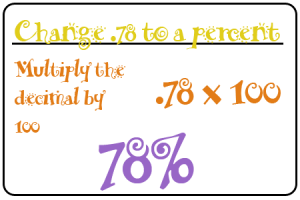Select Page

The Math Movie Network is back with another tutorial! This week, we’ve been learning all about how to find a percent. A percent means a part of or out of 100. It’s essentially another way to represent a fraction that has a denominator of 100.We’ve been learning about the relationship between decimals and percents. We’ve learned that you can change any decimal to a percent by multiplying it by 100.We’ve also learned about how to find a percent based on a fraction. We found that if you divide your numerator by your denominator, then multiply your answer by 100 (move your decimal over two places), then you end up with your percent! Watch below as Wes, Yana, Fiona, and Morgan share how to find a percent from a decimal and a fraction.

*Did this video help you to understand how to find a percent?*

*Where are some places where you might see a percent?*

## Subscribe By Email

Get a weekly email of all new posts.